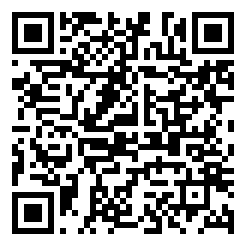Jimmy Zhang Sep 01, 2017
•1. 此处身份证指的是中华人民共和国公民身份证，其它证件不在本文讨论范围之内；
2. 本文大部分是搬运的，别打我……（逃

1. 地址码（6 位）：公民常住户口所在县（市、镇、区）的行政区划代码。
2. 出生日期码（8 位）：公历年、月、日；
3. 顺序码（3 位）：给同地址码同出生日期码的人编订顺序号，其中奇数分配给男性，偶数分配给女性
4. 校验码（1 位）：采用 ISO 7064:1983,MOD 11-2 校验码系统。校验码为一位数字或 X（校验码为 10 的情况）；

1. 将身份证号从右至左排列一词标记为 $a_1$, $a_2$, … ,$a_{18}$，其中 $a_1$ 就是校验码；
2. 分别计算出每一位的权重 $W_i = 2^{i - 1} \ mod \ 11$，得到：
$i$181716151413121110987654321
$Wi$79105842163791058421
3. 计算：$S = \sum\limits_{i=2}^{18} a_i \cdot W_i$
4. 校验码：$a_1 = (12 - (S \ mod \ 11)) \ mod \ 11$

#include "stdio.h"
#include "stdbool.h"
#include "string.h"
#include "math.h"

_Bool validateId(char id)
{
int checksum, sum = 0;
for (int i = 0; i < 17; i++)
{
sum += ((int)pow(2, 17 - i) % 11) * ((int)id[i] - 48);
}
checksum = (12 - (sum % 11)) % 11;
return (int)id == 88 ? checksum == 10 : checksum == (int)id - 48; // 88 == "X"
}

int main()
{
char id;
scanf("%s", id);
printf("%d", validateId(id));
return 0;
}

This blog is under a CC BY-NC-SA 4.0 Unported License.